# A Novel Face Recognition Algorithm for Imbalanced Small Samples

A Novel Face Recognition Algorithm for Imbalanced Small Samples
College of Electronic Information Engineering, Xi’an Technological University, Xi’an 710021, China

Science and Technology on Electromechanical Dynamic Control Laboratory, Xi’an 710065, China

Corresponding Author Email:
masha0443@163.com
Page:
https://doi.org/10.18280/ts.370309
|
DOI:
425-432
28 January 2020
|
Accepted:
10 April 2020
|
Published:
30 June 2020
| Citation

OPEN ACCESS

Abstract:

Deep learning (DL) has become a hotspot in the research of image recognition. However, the DL strategy must be trained with lots of samples that are distributed evenly across classes, i.e. subjected to balanced distribution. Therefore, this paper attempts to design a method to satisfactorily recognize faces in imbalanced small samples. Firstly, the deep convolutional generative adversarial network (DCGAN) was improved to generate data samples with similar distribution as the original training data, creating a balanced training set of sufficient labelled samples. Then, transfer learning was performed to transform the AlexNet, which is pretrained on big dataset, to the balanced target dataset of small samples. Next, the previous convolutional layer was frozen as a feature extractor, and the truncated normal distribution was reinitialized for the next fully-connected layer. Simulations on face recognition show that our method achieved higher recognition rate and less serious overfitting than ordinary CNNs.

Keywords:

feature extraction, face recognition, convolutional neural network (CNN), imbalanced small samples

1. Introduction

Face recognition, a challenging task in computer vision and machine learning (ML), has attracted extensive attention from researchers. Effective face recognition techniques have broad application prospects in various fields, such as national defense, video surveillance, human-computer interaction, and video indexing . However, it is very difficult to achieve high-precision face recognition, owing to numerous unpredictable changes in real life.

With the development of computer technology, image processing and ML have been widely applied to face recognition . For example, Perlibakas  acquired low-dimensional facial features through highly adaptable principal component analysis (PCA), and recognized faces based on distance measurement. However, the recognition became very inaccurate under significant changes of illumination and posture. Luo et al.  combined wavelet transform (WT) and support vector machine (SVM) for face recognition: the wavelet decomposition of low-frequency sub-band coefficients were taken as input features of the SVM. But the combined method brings about a heavy computing load. Zhang et al.  proposed a facial feature extraction method based on the combination of sub-image features: First, the original face image was divided into sub-images; then, each sub-image was subjected to discrete cosine transform, and represented by the maximum coefficient; after that, the coefficients of all sub-images were combined into a vector to represent the features of the entire image; finally, the backpropagation neural network (BPNN) was selected as the classifier. Tan et al.  fused global and local features for face recognition, and achieved a higher recognition rate than single feature extraction methods.

Feature extraction directly affects the precision of the above ML-based face recognition methods. Nonetheless, high-quality feature extraction requires a large amount of prior knowledge. If the face background is complex or subjected to unpredictable changes, the accuracy of face recognition will be greatly suppressed. To make matters worse, the above methods generally adopt shallow algorithms, which are difficult to express complex functions under a limited number of training samples and computing units . In addition, it consumes a lot of time to construct new handcrafted features, not to mention achieving obvious results.

In recent years, computing power, big data and algorithm have been progressing rapidly. As a result, deep learning (DL) has permeated into fields like image detection , image recognition  and speech recognition , promoting the application of computer vision in images and videos. In 1998, Lecun et al.  proposed a convolutional neural network (CNN) called LeNet-5, and successfully applied it to digital recognition, marking a milestone in the development of modern CNNs. In 2012, Krizhevsky et al.  won the ImageNet competition with his deep CNN named AlexNet, which is an iconic model in the CNN field. AlexNet is much faster than LeNet-5 in computing speed, thanks to its 8-layer structure and two parallel graphics processing units (GPUs). Later, Simonyan and Zisserman  designed an even deeper CNN: the VGG model. Szegedy et al.  optimizing the structure of inception modules to densify local sparse vectors. Szegedy’s strategy widens the CNN instead of deepening the network.

The advancement of CNN models is accompanied by the growing popularity of DL-based face recognition . DL techniques have greatly promoted the effect of face recognition. The early studies mainly concentrated on recognizing faces in visible light images with deep networks. Balaban  briefly reviewed DL techniques and representation learning in face recognition, and compared several popular CNNs based on deep models. Sepas-Moghaddam et al.  summarized face recognition solutions based on a new, more encompassing and richer multi-level taxonomy. Unlike traditional ML-based image recognition algorithms, the CNNs support the self-learning of the two-dimensional (2D) spatial correlations in images, eliminating the need to manually extract image features. But the CNNs need to be trained with lots of data samples. If the samples are insufficient, the CNNs will be prone to divergence and overfitting .

The DL has kicked off a revolution in the field of computer vision. Compared with traditional methods, DL-based method trains the model with massive data, enabling the model to extract generalized facial features . In real-world scenarios, however, the numerous data samples are often small in size and clustered in a few of the many classes, that is, the dataset is highly imbalanced. The imbalanced datasets cannot be classified effectively by traditional ML algorithms, which are biased to negative samples.

Currently, imbalanced datasets are mainly classified from three perspectives: data level, algorithm level, and data level plus algorithm level (hybrid level). The data-level approach carries out resampling to change the distribution of the training set and reduce the IR (Imbalanced Rate) between classes, making the training set more balanced, and then classifies the training samples by traditional method. The algorithm-level approach improves the classification algorithm to lower the error that favors negative samples and enhance the recognition rate of positive samples. Fruitful results have been achieved by this approach, despite the difficulty in determining the suitable cost factor matrix in practical problems . The hybrid-level approach inherits the merits of the first two strategies in classification, while resolving their weaknesses [21, 22].

This paper adopts the data-level approach to learn imbalanced small samples. The imbalanced training set comes from the mixture of new samples and original samples. The recognition model for the original sample set might not be able to recognize the new samples in the mixed sample set . As mentioned above, resampling is the key step in the data-level approach. However, the simple random resampling has various shortcomings. To solve the problem, synthetic minority over-sampling technique (SMOTE) comes into being. But the SMOTE focuses on the local neighborhood of the sample point, failing to consider the overall distribution of the dataset. An ideal data-level method for imbalanced learning should directly sample from the data distribution and balance the training set. The generative adversarial network (GAN) lays the basis for building such a method. Proposed by Goodfellow in 2014, the GAN trains the network with an internal adversarial mechanism [24, 25]. The GAN has attracted much attention from the academia and the industry, for its excellent effect arising from the learning of actual data distribution.

In the light of the above, this paper aims to develop a targeted and time-efficient method to extract features from imbalanced small samples, while preventing overfitting. To this end, the deep convolutional GAN (DCGAN) was improved to simulate the data distribution, and to generate face images similar to the training data, making the samples more diverse and the recognition rate immune to sample imbalance. Next, the number of output layer nodes was finetuned by layer freezing method and transfer learning algorithm, aiming to complete the training to the target dataset. During the training, exponential decay of learning rate, L2 regularization, and Adam optimization were adopted separately to enhance the effect of face recognition.

2. Design of Face Recognition Model

2.1 Model design based on transfer learning

The CNN-based image recognition can achieve a high recognition rate, if the recognition model is trained by a large number of labelled samples. But it is very costly to obtain so many labelled data.

Considering the correlation between most data, transfer learning has been widely used to recognize small samples in computer vision tasks. During transfer learning, the model learns dataset A and dataset B in turn. If the two datasets have many similar features, the model will have a good effect after the learning process.

Based on transfer learning and CNN, this paper extends the AlexNet, pretrained by ImageNet dataset, into a small sample model for face recognition in a new target dataset. As shown in Figure 1, the proposed model is implemented in the following steps:

Step 1. Since the pretrained AlexNet is good at feature extraction, the weights of the convolutional layer in the front of AlexNet were frozen, and taken as the feature extractor of the target dataset.

Step 2. The number of finetuned output layer nodes was set as the number of classes in the target dataset.

Step 3. The truncated normal distribution was initialized for the last three fully-connected layers in the pretrained AlexNet, so that the output of each layer has the same distribution value.

Step 4. The parameters of the fully-connected layers were trained and learned on the target dataset, making the model adapt to the target task.

## 1.png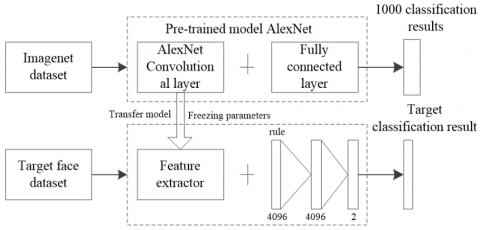Figure 1. Small sample model based on transfer learning

2.1.1 Selecting the activation function for fully-connected layers

In early shallow neural networks, the nonlinear function sigmoid is often adopted as the activation function. During error backpropagation, there are not many intermediate layers. The calculation accuracy can be guaranteed as the error gradient passes through the rear layers. With the growing number of network layers, however, the vanishing gradient problem may occur, using the sigmoid function. The sigmoid function and its backpropagation derivative are illustrated in Figure 2 below.

## 2.png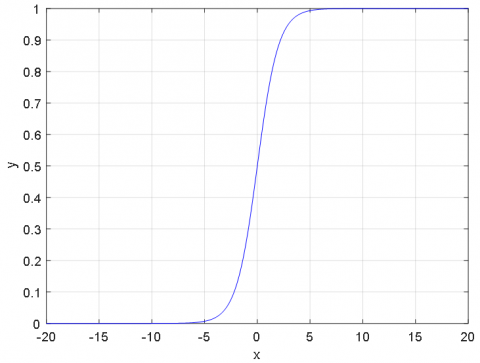(a) Sigmoid function

## 2b.png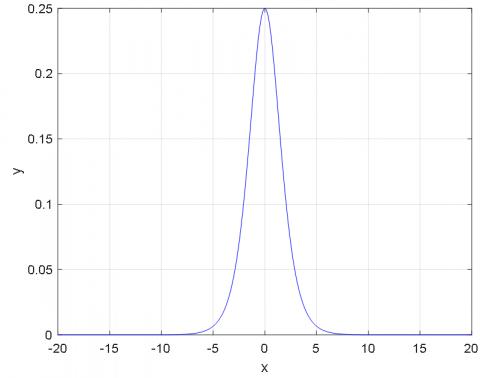(b) Backpropagation derivative

Figure 2. Sigmoid as the activation function

As shown in Figure 2, the gradient of sigmoid function only peaked at 0.25. In deep CNNs (DCNNs), the update gradient of each layer depends on the backpropagation derivative of the activation function. Hence, the amount of parameter update in the rear layers is negatively correlated with that in the front layers. If the amount is too high in the rear layers, the vanishing gradient problem will occur, undermining the network effect. With a constant, nonnegative gradient interval, the rectified linear units (ReLU) was selected as the activation function for nonlinear mapping, which eliminates the problem of vanishing gradient.

2.1.2 L2 regularization

During the training of an ML model, the error of the training set is usually on the decline. Nevertheless, many DCNNs face a common problem: the good performance on the training set does not necessarily lead to a strong generalization ability on unknown input data. The main reason is that, when its capacity surpasses that required by the task, the model will have a good memory of the random noise distribution in each training set, resulting in overfitting. This paper resorts to L2 regularization to prevent overfitting.

L2 regularization adds a penalty term to the loss function. Let L(w) be the loss function of the model on the training set, where w is the set of all parameters in the DCNN. After optimization, the objective function is modified into Lw+λ||w||2, where λ||w||2 is the added penalty term depending on the complexity of the task, and λ is a user-defined hyperparameter controlling the preference for small norm weights. To minimize Lw+λ||w||2, it is necessary to balance the small norm weights and fit the training data. The noise content in the training set can be reduced by limiting the weights, which in turn lowers the chance of overfitting and improves the numerical stability. Therefore, this paper takes the objective function with the regularized penalty function as the loss function for model training.

During error backpropagation, CNNs mainly rely on gradient descent algorithm to update the weights and offsets of each layer:

${{w}_{ij(k\text{+1})}}={{w}_{ij(k)}}+\Delta {{w}_{ij}}={{w}_{ij(k)}}-\eta {{g}_{\text{k}}}$             (1)

where, wij(k) and wij(k+1) are the current weight and updated weight, respectively; η is the learning rate; gk is the current gradient.

For DCNNs, however, the updated weights differ in the dependency on the objective function. Even if the algorithm has reached the minimum value, some weights still have a large gradient. Therefore, the Adam optimization was introduced to set different learning rates for different weights of the fully-connected layers. Through the optimization, the weights were adjusted adaptively throughout the learning. The change of each weight depends on the weighted average of its own momentum and the cumulative square gradient, ensuring that the weights are adapted to different learning rates.

2.2 Model design for imbalanced samples

In many cases, the training samples are not distributed in a balanced manner. Instead, some classes in the training set have greater weights than others, i.e. more samples are clustered in these classes. The imbalanced samples often lead to a low confidence in the recognition rate.

## 3.png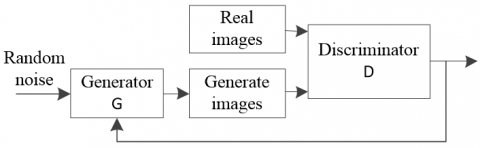Figure 3. The GAN model

To overcome the problem of imbalanced samples, this paper modifies the samples with the GAN. This unsupervised learning algorithm can generate samples with a similar distribution as the training data, and expand the size of a small number of labelled samples. As shown in Figure 3, the GAN model is composed of a generator G and a discriminator D. Based on the input random noise, the generator simulates the distribution of real images, and generates new images; the two types of images are both inputted into the discriminator, which will judge which of them is generated and which is real.

During the training, the generator aims to promotes the accuracy of the discriminator, while the discriminator aims to make correct judgements and labelling of the generated and real images. The training process is to find a Nash equilibrium between the generator and discriminator. The optimization objectives of the discriminator and generator can be respectively expressed as:

$L(D)\text{=}\log ({{D}_{1}}(x))+\log (1-{{D}_{2}}(G(z)))$           (2)

$L(G)=\log ({{D}_{2}}(G(z)))$           (3)

where, x is the set of real images; z is the random noise; D1(x) is the probability that the discriminator makes a correct judgement of a real image; D2(G(z)) is the probability that the discriminator makes a correct judgement of a generated image. The sum of formulas (2) and (3) is the objective function of the GAN model.

## 4.pngFigure 4. The structure of the generator

## 5.png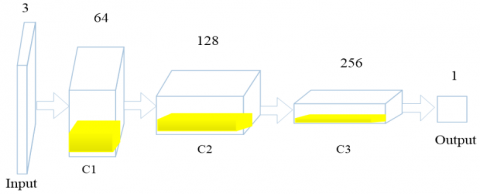Figure 5. The structure of the discriminator

Nonetheless, the images generated by the traditional GAN model often lack diversity, which undermines the model performance. To solve the problem, the DCGAN was improved to estimate the potential distribution of samples and generate new samples. In the improved DCGAN, the generator uses ReLU as the activation function, and learns spatial up-sampling through micro-step full deconvolution; the discriminator uses LeakyReLU as the activation function, and replaces spatial pooling and spatial down-sampling through convolution with a preset step size. The generator and discriminator in the improved DCGAN are respectively depicted in Figures 4 and 5, where DC and C are transposed convolution and convolution, respectively.

As shown in Figure 4, the transposed convolution is the main operation in the generator model. Except the tanh in the output layer, the ReLU was adopted in the other layers. In addition, the generator consists of an input layer, three transposed convolution layers (DC1-3) and an output layer.

The deeper the network depth, the larger the image size. Firstly, a 100-dimensional random noise is imported to the input layer. In the DC1 layer, the random noise is passed through 256 * 8 * 8 nodes, which are fully connected to the input layer, and then mapped into a 256 * 8 * 8 feature map. In the DC2 layer, the feature map is adjusted to 16 * 16 * 128 through transposed convolution by 128 convolution kernels of the size 5 * 5, and ReLU activation function with a step size of 2 under the SAME mode. In the DC3, the feature map is further adjusted to 32 * 32 * 64 through transposed convolution by 64 convolution kernels of the size 5 * 5, and ReLU activation function with a step size of 2 under the SAME mode. To output a three-cannel color image, the depth of the output layer was set to 3. The feature image from DC3 layer is deconvoluted into a 64 * 64 * 3 output image.

As shown in Figure 5, convolution is the main operation in the discriminator model. The deeper the network depth, the larger the image size. The size of feature map was designed symmetrical to that of the generator. LeakyReLU was adopted as the activation function of all the layers.

The deeper the network depth, the smaller the image size. Firstly, a 64 * 64 * 3 color image is imported, and transformed into a feature map C1 of 32 * 32 * 64 through 64 SAME convolutions, using 5 * 5 convolution kernels and a step size of 2. C1 is further transformed into a feature map C2 of 16 * 16 * 128 through 128 SAME convolutions, using 5 * 5 convolution kernels and a step size of 2. Similarly, C2 is transformed into a feature map C3 of 8 * 8 * 256 through 256 SAME convolutions, using 5 * 5 convolution kernels and a step size of 2. The output layer has only 1 node. If C3 is considered as a real image, the output layer will output 1; if C3 is considered as a generated image, the output layer will output 0.

2.3 Model design for imbalanced small samples

To solve the imbalance of target training set, this paper improves the DCGAN to generate face images with similar distribution as the original dataset, and expands the samples to obtain a balanced training set. Then, the transfer learning was adopted to migrate the pretrained AlexNet model and ImageNet parameter to the balanced training set. On this basis, the previous convolutional layer was frozen as a feature extractor, and the truncated normal distribution was reinitialized for the next fully-connected layer. In addition, the size of the output layer was adjusted to the number of classes of the target dataset. Figure 6 illustrates the designed face recognition model.

## 6.png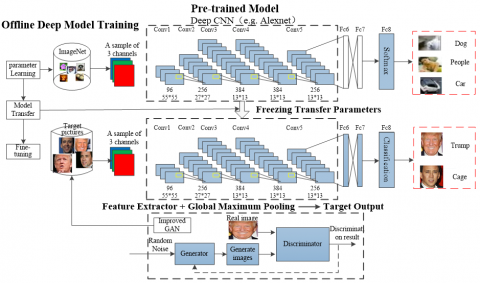Figure 6. Face recognition model

3. Simulations and Results Analysis

The simulation data were downloaded from GitHub, which contains two types of face images, namely, 318 images in dataset A and 376 images in dataset B. Figure 7 shows part of the sample datasets used in our simulations. The simulations were carried out on TensorFlow under Windows 10 (64bit), using an Intel® Core™ i7-9700K Processor (3.60GHz), a memory of 64.0GB, and a GeForce RTX 2080 Ti graphic card.

## 7.pngFigure 7. Part of the sample datasets

3.1 Improved DCGAN on imbalanced samples

## 8.png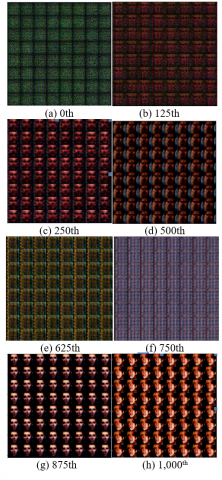Figure 8. The feature maps generated by the improved DCGAN in different iterations of generator training

The improved DCGAN was trained on datasets A and B, respectively. Then, the trained generator was used to generate images. Figure 8 above presents the feature maps generated from a batch of images in different iterations of the generator training. It can be seen that the facial features learned by the improved DCGAN were initially chaotic points. Then, forehead and eye features were gradually learned, followed by some low-level global features in the middle. After that, some high-level texture features were learned. Finally, the low-level and intermediate-level features were synthetized into high-level facial features.

3.2 Face recognition of improved DCGAN

Before training, the original face images were normalized to the same size 227*227, which is the size of the input image to the AlexNet. Then, the face dataset was trained through transfer learning. The weights of the simulation were initialized by truncated normal distribution method. Then, the Adam optimization was performed to adaptively update image batches. The batch size was set to 32. Besides, the learning rate decayed exponentially in reverse update. To control overfitting, the loss function was subjected to L2 regularization. The training parameters are listed in Table 1.

Table 1. The training parameters

 Name Value Training set ratio 0.8 Test set ratio 0.2 Pooling method Max pooling Activation function ReLU Loss function Cross entropy Optimization algorithm Adam Learning rate 0.001 Classifier softmax Dropout 0.5 Number of iterations 3,500

## 9.png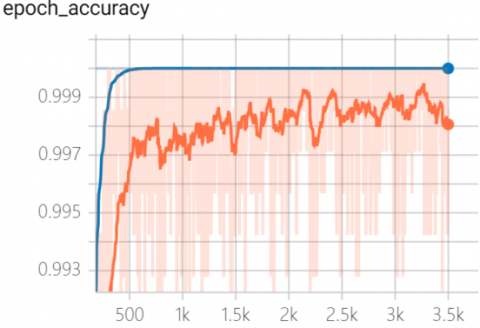Figure 9. Comparison of recognition rates between training set and test set

## 10.pngFigure 10. Comparison of loss values between training set and test set

Once the weights obeyed truncated normal distribution, the improved DCGAN was trained and tested. Figure 9 compares the recognition rates between the training set and the test set after 3,500 iterations. Figure 10 compares the loss values between the two sets. In both figures, the curve of the training set is in red and that of the test set is in blue. Obviously, the improved DCGAN started to converge at around 750 iterations. The recognition rates of the training set and the test set were both around 99%, while the losses of the two sets were very low (<0.001).

3.3 Improved DCGAN on original imbalanced small samples

## 11.pngFigure 11. Comparison of recognition rates and loss values between training set and test set on the original imbalanced small samples

Furthermore, all layers of the AlexNet were reinitialized, and trained on the original imbalanced small samples and the balanced set generated by the improved DCGAN. The training parameters were configured the same as Table 1. Figure 11 compares the recognition rates and loss values between the training set and the test set of the reinitialized AlexNet on the original imbalanced small samples; Figure 12 compares the recognition rates and loss values between the training set and the test set of the reinitialized AlexNet on the balanced set generated by the improved DCGAN. In both figures, the curve of the training set is in red and that of the test set is in blue.

As shown in Figure 11, on the original imbalanced small samples, the recognition rates on the training set and test set converged at the same time during the training. At iteration 1,500, the recognition rate on the training set gradually approached 1, while that on the test set was about 93%. However, the loss value on the test set increased over time, indicating that the network diverged and faced serious overfitting.

The performance of the reinitialized AlexNet was constrasted with that of the imrpoved DCGAN in Table 2.

Table 2. Comparison of simulation results

 Dataset Recognition rate Loss value (Original training set, test set) (1, 0.93) Fluctuation, nonconvergence (Balanced training set, test set) (0.95, 0.88) (0.1, 0.35) Transfer learning + DCGAN (0.999, 0.99) (0.001, 0.005)

## 12.png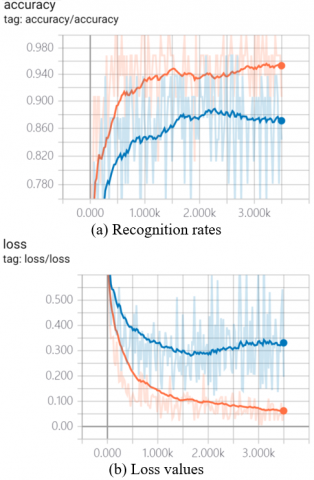Figure 12. Comparison of recognition rates and loss values between training set and test set on the balanced set generated by the improved DCGAN

As shown in Figure 12, on the balanced set generated by the improved DCGAN, the recognition rates on the training set and test set converged before 2,500 iterations. The recognition rate of the training set was about 95%, and that of the test set was about 88%. By contrast, after 2,500 iterations, the loss values on the two sets began to increase, resulting in overfitting. Of course, the loss value curves were smoother than those on the original dataset.

The comparison shows that the balanced set generated by the improved DCGAN is superior to the imbalanced sample dataset. However, overfitting is not fully eliminated due to the depth of the network.

4. Conclusion

The CNN-based image recognition does not need to extract features manually. But this approach cannot achieve a high recognition rate unless the recognition model is trained by a large number of labelled samples. Therefore, this paper proposed a face recognition method for imbalanced small samples, based on hybrid supervised learning networks. Firstly, the DCGAN was improved to generate face images with similar distribution as the training data, making the samples more diverse and the recognition rate immune to sample imbalance. After that, the number of output layer nodes was finetuned by layer freezing method and transfer learning algorithm, such as to complete the training to the target dataset. Then, exponential decay of learning rate, L2 regularization, and Adam optimization were adopted in the training process. Simulation results show that our method prevents the CNN from divergence in the presence of small samples, and enables the network to converge to the optimal solution. Our method achieved a high recognition rate (0.9251) on popular face datasets.

Acknowledgment

This work is supported by Science and Technology Laboratory on Electromechanical Dynamic Control (Grant No.: 6142601200301), National Key Research and Development Program (Grant No.: 2016YFE0111900), Shaanxi International Science and Technology Cooperation Program (Grant No.: 2020GY-176 and 2018KW-022), and Independent Intelligent Control Research and Innovation Team.

References

 Zou, G.F., Fu, G.X. Li, H.T., Gao, M.L. (2015). A Survey of Multi-pose Face Recognition. Pattern Recognition and Artificial Intelligence, 28(7): 613-625. https://doi.org/10.16451/j.cnki.issn1003-6059.201507005

 Kremer, J., Stensbo-Smidt, K., Gieseke, F., Pedersen, K. S., Igel, C. (2017). Big universe, big data: machine learning and image analysis for astronomy. IEEE Intelligent Systems, 32(2): 16-22. https://doi.org/10.1109/MIS.2017.40

 Perlibakas, V. (2004). Distance measures for PCA-based face recognition. Pattern Recognition Letters, 25(6): 711-724. https://doi.org/10.1016/j.patrec.2004.01.011

 Luo, B., Zhang, Y., Pan, Y.H. (2005, June). Face recognition based on wavelet transform and SVM. In 2005 IEEE International Conference on Information Acquisition, pp. 8977177. https://doi.org/10.1109/ICIA.2005.1635115

 Zhang, J., Cheng, F.H., Lin, X.M., Li, R., Wang, S. (2007). Research on Face Recognition Technology Based on Sub-Image Feature Combination. Journal of Hunan University (Natural Sciences), 16(6): 70-73.

 Tan, H., Yang, B., Ma, Z. (2013). Face recognition based on the fusion of global and local HOG features of face images. IET Computer Vision, 8(3): 224-234. https://doi.org/10.1049/iet-cvi.2012.0302

 Braverman, M. (2011). Poly-logarithmic independence fools bounded-depth boolean circuits. Communications of the ACM, 54(4): 108-115. https://doi.org/10.1145/1924421.1924446

 Teki, S.M., Varma, M.K., Yadav, A.K. (2019). Brain tumour segmentation using U-net based adversarial networks. Traitement du Signal, 36(4): 353-359. https://doi.org/10.18280/ts.360408

 Hinton, G., Deng, L., Yu, D., George, E.D., Abdel-rahman, M., Navdeep, J., Andrew, S., Vincent, V., Patrick, N., Tara, N.S., Brian, K. (2012). Deep Neural Networks for Acoustic Modeling in Speech Recognition: The Shared Views of Four Research Groups. IEEE Signal Processing Magazine, 29(6): 82-97. https://doi.org/10.1109/MSP.2012.2205597

 Noda, K., Yamaguchi, Y., Nakadai, K., Okuno, H.G., Ogata, T. (2015). Audio-visual speech recognition using deep learning. Applied Intelligence, 42(4): 722-737. https://doi.org/10.1007/s10489-014-0629-7

 Lecun, Y., Bottou, L., Bengio, Y., Haffner, P. (1998). Gradient-based learning applied to document recognition. Proceedings of the IEEE, 86(11): 2278-2324. https://doi.org/10.1109/5.726791

 Krizhevsky, A., Sutskever, I., Hinton, G.E. (2012). Imagenet classification with deep convolutional neural networks. In Advances in Neural Information Processing Systems, 1097-1105. https://doi.org/10.1145/3065386

 Simonyan, K., Zisserman, A. (2014). Very deep convolutional networks for large-scale image recognition. arXiv preprint arXiv:1409.1556.

 Szegedy, C., Liu, W., Jia, Y., Sermanet, P., Reed, S., Anguelov, D., Rabinovich, A. (2015). Going deeper with convolutions. In Proceedings of the IEEE Conference on Computer Vision and Pattern Recognition, pp. 1-9. https://doi.org/10.1109/CVPR.2015.7298594

 Sun, X., Wu, P., Hoi, S. C. (2018). Face detection using deep learning: An improved faster RCNN approach. Neurocomputing, 299: 42-50. https://doi.org/10.1016/j.neucom.2018.03.030

 Balaban, S. (2015). Deep learning and face recognition: the state of the art. In Biometric and Surveillance Technology for Human and Activity Identification XII, 9457: 94570B. https://doi.org/10.1117/12.2181526

 Sepas-Moghaddam, A., Pereira, F.M., Correia, P.L. (2019). Face recognition: A novel multi-level taxonomy based survey. IET Biometrics, 9(2): 58-67. https://doi.org/10.1049/iet-bmt.2019.0001

 Tao, Q.Q., Zhan, S., Li, X.H., Kurihara, T. (2016). Robust face detection using local CNN and SVM based on kernel combination. Neurocomputing, 211: 98-105. https://doi.org/10.1016/j.neucom.2015.10.139

 Ferrari, C., Lisanti, G., Berretti, S., Del Bimbo, A. (2017). Investigating nuisance factors in face recognition with DCNN representation. In Proceedings of the IEEE Conference on Computer Vision and Pattern Recognition Workshops, pp. 81-89. https://doi.org/10.1109/CVPRW.2017.86

 Srivastava, N., Hinton, G., Krizhevsky, A., Sutskever, I., Salakhutdinov, R. (2014). Dropout: A simple way to prevent neural networks from overfitting. The Journal of Machine Learning Research, 15(1): 1929-1958.

 Sun, Y., Chen, Y., Wang, X., Tang, X. (2014). Deep learning face representation by joint identification-verification. In Advances in Neural Information Processing Systems, 2014: 1988-1996.

 Khan, S.H., Hayat, M., Bennamoun, M., Sohel, F.A., Togneri, R. (2017). Cost-sensitive learning of deep feature representations from imbalanced data. IEEE Transactions on Neural Networks and Learning Systems, 29(8): 3573-3587. https://doi.org/10.1109/TNNLS.2017.2732482

 Song, X., Gao, S., Chen, C.B., Cao, K., Huang, J. (2018). A new hybrid method in global dynamic path planning of mobile robot. International Journal of Computers Communications & Control, 13(6): 1032-1046. https://doi.org/10.15837/ijccc.2018.6.3153.

 Goodfellow, I.J., Pouget–Abadie, J., Mirza, M., Xu, B., Warde-Farley, D., Ozair, S., Courville, A., Bengio, Y. (2014). Generative adversarial networks. Advances in Neural Information Processing Systems, 3: 2672-2680.

 Song, X., Chen, H., Xue, Y. (2015). Stabilization precision control methods of photoelectric aim-stabilized system. Optics Communications, 351: 115-120. https://doi.org/10.1016/j.optcom.2015.04.056